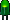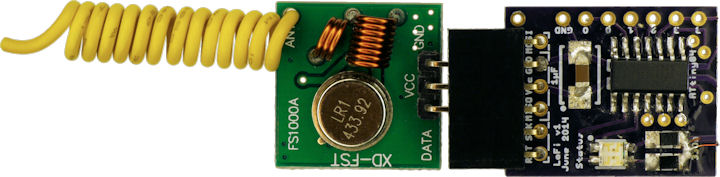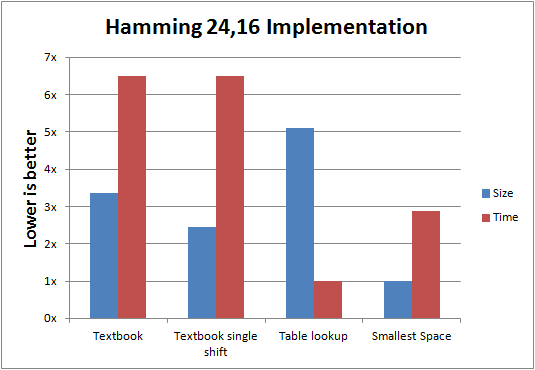• Home• Patreon• Links• Books

# Hamming Error Correcting Code (ECC)

Hamming error-correcting code (ECC) is a method of both detecting and correcting errors in data. It can be used for storage and transmission integrity.

Error correcting codes work by mathematically combining data values, such that if the math isn’t equal when you look at it later, something changed.

For example, imagine you wanted to transmit the following values: 7, 4, 5. Add together the first two values (=11) and add together the last two values (=9) and transmit all of that data: 7, 4, 5, 11, 9.

Oh no! The receiver received 8, 4, 5, 11, 9. It performs the ECC math and gets 12 and 9. It knows something is wrong with the first value, because the second and third values still add up to 9. The correct first value can be determined by reversing the math: 11 - 4 = 7. So, not only did the receiver detect the error, but it corrected it as well.

Hamming works on a smaller resolution, the bit, but still follows the practice of combining values together.

All error correcting codes have a limit to how many errors they can detect and how many they can correct. In the example above, if the data had been received as 8, 4, 6, 11, 9 (two errors), the errors would be detected but uncorrectable. There is no way to tell whether the actual data was 8, 3, 6, 11, 9 or 7, 4, 5, 11, 9.

Error correcting codes increase the total amount of information that must be transmitted or stored. Therefore, you have to make a decision about whether there is enough of a risk of data corruption.

## Real World Example

I am currently entered in a Hack A Day contest where the goal is create a 'connected' device. I decided to make a really inexpensive data delivery module (LoFi) that transmits information from appliances and project throughout the home. I needed the cheapest transmitter I could buy, which meant that it wasn’t particularly robust.LoFi Sender (the green board is the off-the-shelf transmitter)

To detect and correct transmission errors, I selected the Hamming 12,8 algorithm. That means for every 12 bits, 8 of them are for data and 4 for the correcting code. Unfortunately, 12 is a slightly awkward size. A multiple of 8 bits would be easier. Doubling the algorithm to 24,16 means that for every three bytes (24 = 3 * 8 bits), it delivers two bytes of data (16 = 2 * 8 bits), and one byte of ECC.

In both cases, that’s 50% overhead. Hamming can be much more efficient than this, but the program needs to run on a relatively slow microcontroller with limited program space. Therefore, this is a compromise.

## Textbook Code Snippet

The textbook sample code is as follows:

```#define BitToBool(byte, n) ((byte>>(n-1)) & 1)

// Given two bytes to transmit, this returns the parity
// as a byte with the lower nibble being for the first byte,
// and the upper nibble being for the second byte.
byte DL_HammingCalculateParity2416(byte first, byte second)
{
// This is the textbook way to calculate hamming parity.
return ((BitToBool(first, 1) ^ BitToBool(first, 2) ^ BitToBool(first, 4) ^ BitToBool(first, 5) ^ BitToBool(first, 7))) +
((BitToBool(first, 1) ^ BitToBool(first, 3) ^ BitToBool(first, 4) ^ BitToBool(first, 6) ^ BitToBool(first, 7))<<1) +
((BitToBool(first, 2) ^ BitToBool(first, 3) ^ BitToBool(first, 4) ^ BitToBool(first, 8))<<2) +
((BitToBool(first, 5) ^ BitToBool(first, 6) ^ BitToBool(first, 7) ^ BitToBool(first, 8))<<3) +

((BitToBool(second, 1) ^ BitToBool(second, 2) ^ BitToBool(second, 4) ^ BitToBool(second, 5) ^ BitToBool(second, 7))<<4) +
((BitToBool(second, 1) ^ BitToBool(second, 3) ^ BitToBool(second, 4) ^ BitToBool(second, 6) ^ BitToBool(second, 7))<<5) +
((BitToBool(second, 2) ^ BitToBool(second, 3) ^ BitToBool(second, 4) ^ BitToBool(second, 8))<<6) +
((BitToBool(second, 5) ^ BitToBool(second, 6) ^ BitToBool(second, 7) ^ BitToBool(second, 8))<<7);
}
```

Although it is only a dozen lines of source code, there is a lot of bit logic and bit shifting going on. In fact, when the software is compiled, it takes 19 seconds to compute 65536 values at 1 MHz on an Atmel ATtiny84. Wow! That’s slow.

Sanity check:
(1,000,000 Hz * 19 seconds) / 65536 ECCs to compute = 290 MCU cycles per ECC.
The subroutine consumes 185 bytes, so that’s in the ballpark of being an accurate measurement considering overhead and that not every MCU instruction will complete in one cycle.

The cause of the slowness is that the Atmel AVR microcontroller does not support multiple bit shifts per instruction. Put another way, to shift a bit twice, two instructions are needed. I absolutely cannot fathom why a product whose target audience is bit pushers would lack multi-shift instructions.

## Hamming Lookup Table

The fastest solution is to precompute all of the ECC byte values and store them in a table. Here is the Hamming 8,4 table:

```// For processors with a Harvard architecture where you want
// to indicate the table should be in program space rather than RAM.
// On other processors, just define __flash as blank.
#ifndef __flash
#define __flash
#endif

// This contains all of the precalculated parity values for a byte (8 bits).
// This is very fast, but takes up more program space than calculating on the fly.
__flash byte _hammingCalculateParityFast128=
{
0,  3,  5,  6,  6,  5,  3,  0,  7,  4,  2,  1,  1,  2,  4,  7,
9, 10, 12, 15, 15, 12, 10,  9, 14, 13, 11,  8,  8, 11, 13, 14,
10,  9, 15, 12, 12, 15,  9, 10, 13, 14,  8, 11, 11,  8, 14, 13,
3,  0,  6,  5,  5,  6,  0,  3,  4,  7,  1,  2,  2,  1,  7,  4,
11,  8, 14, 13, 13, 14,  8, 11, 12, 15,  9, 10, 10,  9, 15, 12,
2,  1,  7,  4,  4,  7,  1,  2,  5,  6,  0,  3,  3,  0,  6,  5,
1,  2,  4,  7,  7,  4,  2,  1,  6,  5,  3,  0,  0,  3,  5,  6,
8, 11, 13, 14, 14, 13, 11,  8, 15, 12, 10,  9,  9, 10, 12, 15,
12, 15,  9, 10, 10,  9, 15, 12, 11,  8, 14, 13, 13, 14,  8, 11,
5,  6,  0,  3,  3,  0,  6,  5,  2,  1,  7,  4,  4,  7,  1,  2,
6,  5,  3,  0,  0,  3,  5,  6,  1,  2,  4,  7,  7,  4,  2,  1,
15, 12, 10,  9,  9, 10, 12, 15,  8, 11, 13, 14, 14, 13, 11,  8,
7,  4,  2,  1,  1,  2,  4,  7,  0,  3,  5,  6,  6,  5,  3,  0,
14, 13, 11,  8,  8, 11, 13, 14,  9, 10, 12, 15, 15, 12, 10,  9,
13, 14,  8, 11, 11,  8, 14, 13, 10,  9, 15, 12, 12, 15,  9, 10,
4,  7,  1,  2,  2,  1,  7,  4,  3,  0,  6,  5,  5,  6,  0,  3,
};

// Given a byte to transmit, this returns the parity as a nibble
nibble DL_HammingCalculateParity128(byte value)
{
return _hammingCalculateParityFast128[value];
}

// Given two bytes to transmit, this returns the parity
// as a byte with the lower nibble being for the first byte,
// and the upper nibble being for the second byte.
byte DL_HammingCalculateParity2416(byte first, byte second)
{
return (_hammingCalculateParityFast128[second]<<4) | _hammingCalculateParityFast128[first];
}
```

It takes about 3 seconds to lookup 65536 values. The table lookup is over 6 times faster, but unfortunately it consumes 280 bytes versus 185 bytes for the textbook example.

## Hamming Optimized for 8-Bit Microcontroller

Program space is a premium on low-cost microcontrollers. Every byte that is used can put you over the limit to the next most expensive microcontroller model. Furthermore, every byte used for the Hamming algorithm takes away from other possible features of the product.

Looking at the algorithm from a different perspective, we see that each source bit contributes to multiple checksum (ECC) bits. Since bit shifting is slow on the Atmel AVR, let’s use branch statements and constants to examine and apply bits without shifting.

```// Given a byte to transmit, this returns the parity as a nibble
nibble DL_HammingCalculateParity128(byte value)
{
// Exclusive OR is associative and commutative,
// so order of operations and values does not matter.
nibble parity;

if ( ( value & 1 ) != 0 )
{
parity = 0x3;
}
else
{
parity = 0x0;
}

if ( ( value & 2 ) != 0 )
{
parity ^= 0x5;
}

if ( ( value & 4 ) != 0 )
{
parity ^= 0x6;
}

if ( ( value & 8 ) != 0 )
{
parity ^= 0x7;
}

if ( ( value & 16 ) != 0 )
{
parity ^= 0x9;
}

if ( ( value & 32 ) != 0 )
{
parity ^= 0xA;
}

if ( ( value & 64 ) != 0 )
{
parity ^= 0xB;
}

if ( ( value & 128 ) != 0 )
{
parity ^= 0xC;
}

return parity;
}

// Given two bytes to transmit, this returns the parity
// as a byte with the lower nibble being for the first byte,
// and the upper nibble being for the second byte.
byte DL_HammingCalculateParity2416(byte first, byte second)
{
return (DL_HammingCalculateParity128(second) << 4) | DL_HammingCalculateParity128(first);
}
```

The resulting software is twice as fast as the textbook and uses half the space.

## Results

In the graph below, you can compare the size and speed of implementation variations of the Hamming 24,16 algorithm. (The textbook single shift algorithm was not described in this article as it wasn’t a significant improvement.)Here is the C source code library for Hamming 24,16 error-correcting code (ECC). It includes both the ECC generator, as well as the detection/correction routines.